Processing ......FreeComputerBooks.com Links to Free Computer, Mathematics, Technical Books all over the World

Topology: A Categorical Approach
🌠 Top Free C Programming Books - 100% Free or Open Source
• Title: Topology: A Categorical Approach
• Author(s) Tai-Danae Bradley, Tyler Bryson and John Terilla
• Publisher: The MIT Press (August 18, 2020); eBook (Creative Commons Edition)
• Paperback: 166 pages
• eBook: PDF Files
• Language: English
• ISBN-10: 0262539357
• ISBN-13: 978-0262539357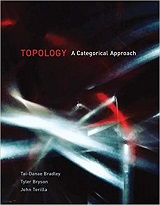Book Description

A graduate-level textbook that presents basic topology from the perspective of category theory.

This graduate-level textbook on topology takes a unique approach: it reintroduces basic, point-set topology from a more modern, categorical perspective. Many graduate students are familiar with the ideas of point-set topology and they are ready to learn something new about them.

Teaching the subject using category theory - a contemporary branch of mathematics that provides a way to represent abstract concepts - both deepens students' understanding of elementary topology and lays a solid foundation for future work in advanced topics.

The book expertly guides students of topology through the important transition from undergraduate student with a solid background in analysis or point-set topology to graduate student preparing to work on contemporary problems in mathematics.

• Tai-Danae Bradley is a PhD candidate in mathematics at the CUNY Graduate Center and the creator of the popular math blog, Math3ma.
• Tyler Bryson is a PhD candidate in mathematics at the CUNY Graduate Center.
• John Terilla is Professor of Mathematics at Queens College and on the Doctoral Faculty at the CUNY Graduate Center.
Reviews, Ratings, and Recommendations: Related Book Categories: Read and Download Links:Similar Books:
•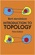Introduction to Topology (Bert Mendelson, et al.)

This concise book offers an ideal introduction to the fundamentals of topology. The book's principal aim is to provide a simple, thorough survey of elementary topics in the study of collections of objects, or sets, that possess a mathematical structure.

•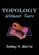Topology without Tears (Sidney A. Morris)

General topology is the branch of topology dealing with the basic set-theoretic definitions and constructions used in topology. The aim of this is to provide a thorough grouding of general topology. It offers an ideal introduction to the fundamentals of topology.

•Topological Groups: Yesterday, Today, Tomorrow (S. A. Morris)

In 1900, David Hilbert asked whether each locally euclidean topological group admits a Lie group structure. This was the fifth of his famous 23 questions which foreshadowed much of the mathematical creativity of the twentieth century.

•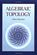Algebraic Topology (Allen Hatcher)

This introductory text is suitable for use in a course on the subject or for self-study, featuring broad coverage and a readable exposition, with many examples and exercises. The four main chapters present the basics: fundamental group and covering spaces, homology and cohomology, higher homotopy groups, and homotopy theory generally.

•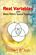Real Variables with Basic Metric Space Topology (Robert B. Ash)

Designed for a first course in real variables, this text presents the fundamentals for more advanced mathematical work, particularly in the areas of complex variables, measure theory, differential equations, functional analysis, and probability.

•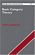Basic Category Theory (Tom Leinster)

Assuming little mathematical background, this short introduction to Category Theory is ideal for beginning graduate students or advanced undergraduates learning category theory for the first time.

•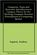Categories, Types, and Structures (Andrea Asperti, et al)

This book introduces Category Theory at a level appropriate for computer scientists and provides practical examples in the context of programming language design. It pursues the more complex mathematical semantics of data types and programs.

•Categorical Homotopy Theory (Emily Riehl)

This book develops abstract homotopy theory from the categorical perspective with a particular focus on examples. It helps consolidate and simplify one's understanding of derived functors, homotopy limits and colimits, and model categories, among others.

•Higher Topos Theory (Jacob Lurie)

This book presents the foundations of Higher Topos Theory, using the language of weak Kan complexes, and shows how existing theorems in algebraic topology can be reformulated and generalized in the theory's new language.

Book Categories
 :All CategoriesTop Free BooksRecent BooksMiscellaneous BooksComputer EngineeringComputer LanguagesComputer ScienceData Science/DatabasesJava and Java EE (J2EE)Linux and UnixMathematicsMicrosoft and .NETMobile ComputingNetworking and CommunicationsSoftware EngineeringSpecial TopicsWeb Programming
Other Categories